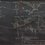# PSA #1

When writing in LaTeX, never ever ever write like this:

$\overset { \rightarrow }{ { F }_{ e } } ={ k }_{ e }\dfrac { { q }_{ 1 }{ q }_{ 2 } }{ { r }^{ 2 } } \overset { \wedge }{ r }$

The above was "expressed" as

\overset { \rightarrow  }{ { F }_{ e } } ={ k }_{ e }\dfrac { { q }_{ 1 }{ q }_{ 2 } }{ { r }^{ 2 } } \overset { \wedge  }{ r }


Well, you might ask, it looks alright to me. Doesn't really matter what the innards are, just as long as the final product is digestible.

WRONG.

The issue with sloppy LaTeX writing is twofold: 1. people cannot learn from your LaTeX; and 2. it is much harder to check your work for mistakes, or to "debug" it. If I wrote

\overset { \rightarrow  }{ { F }_{ e } } ={ k }_{ e }\dfrac { { { q }_{ 1 }{ q }_{ 2 } }{ { r }^{ 2 } } \overset { \wedge  }{ r }


it will not be a valid LaTeX input, and it would take ages to track down the mistake(s), however minute.

Here are a few guidelines to help you become a better LaTeXian/ist/er.

Guideline 1: Don't use spaces unless they are a necessity, around equals signs, or within long expressions to signify terms/sub-expressions.

Compare this:

\overset { \rightarrow  }{ { F }_{ e } } ={ k }_{ e }\dfrac { { { q }_{ 1 }{ q }_{ 2 } }{ { r }^{ 2 } } \overset { \wedge  }{ r }


to this:

\overset{\rightarrow}{{F}_{e}} = {k}_{e} \dfrac{{{q}_{1}{q}_{2}}{{r}^{2}} \overset{\wedge}{r}


Much neater.

(Some would write

\overset{\rightarrow}{{F}_{e}} = {k}_{e}\dfrac{{{q}_{1}{q}_{2}}{{r}^{2}}\overset{\wedge}{r}


instead [notice that spaces have been removed before \dfrac and \overset]; this is a matter of taste. The guideline here is to minimise spacing, essentially.)

Guideline 2: Don't use braces unless they are a necessity.

Compare this:

\overset{\rightarrow}{{F}_{e}} = {k}_{e} \dfrac{{{q}_{1}{q}_{2}}{{r}^{2}} \overset{\wedge}{r}


to this:

\overset{\rightarrow}{F_e} = k_e \dfrac{q_1q_2}{r^2} \overset{\wedge}{r}


Again, a drastic improvement in cleanliness.

Guideline 3: Look for shortcuts/features to make your life easier.

We may use \vec{} and \hat{} rather than the cumbersome \overset{}{} to obtain at once a more concise and illuminating LaTeX input. Compare this:

\overset{\rightarrow}{F_e} = k_e \dfrac{q_1q_2}{r^2} \overset{\wedge}{r}


to this:

\vec{F_e} = k_e \dfrac{q_1q_2}{r^2} \hat{r}


Not only that, $\overset{\wedge}{r}$ and $\hat{r}$ differ in that the latter is surely recognised as the notation for unit vectors, which the former may be confusing to readers.

(This is especially important when it comes to LaTeX formatting, where you can set shortcuts. I personally use \conj{}, \mean{}, etc to mean the conjugate, the sample mean, etc, while in practice they are still equivalent to \overline{}. This is for readability's/checking's sake.)

Now, putting this altogether, we have

$\vec{F_e} = k_e \dfrac{q_1q_2}{r^2} \hat{r}$

which is not only equivalent to what we want, but also elegant, legible and efficient.

Other guidelines:

Stay consistent. For example, stick to the order in which you input _ and ^ (subscript and superscript). Most people do the former first, followed by the latter.

Take care not to make mistakes like $n^10$ instead of $n^{10}$.

[WIP?]

Examples:

{ \int}_{ 0 }^{ \infty  }e^{- x^{  2 }_{ 0 } } \  d x_{ 0}=\frac { \sqrt{ \pi } }{ 2}


vs

\int_0^\infty e^{-x_0^2} \ dx_0 = \frac{\sqrt{\pi}}{2}


[also WIP]

PS If any of the mods touch this I'll skin you. Another friendly PSA by Jake.Note by Jake Lai
5 years, 4 months ago

This discussion board is a place to discuss our Daily Challenges and the math and science related to those challenges. Explanations are more than just a solution — they should explain the steps and thinking strategies that you used to obtain the solution. Comments should further the discussion of math and science.

When posting on Brilliant:

• Use the emojis to react to an explanation, whether you're congratulating a job well done , or just really confused .
• Ask specific questions about the challenge or the steps in somebody's explanation. Well-posed questions can add a lot to the discussion, but posting "I don't understand!" doesn't help anyone.
• Try to contribute something new to the discussion, whether it is an extension, generalization or other idea related to the challenge.

MarkdownAppears as
*italics* or _italics_ italics
**bold** or __bold__ bold
- bulleted- list
• bulleted
• list
1. numbered2. list
1. numbered
2. list
Note: you must add a full line of space before and after lists for them to show up correctly
paragraph 1paragraph 2

paragraph 1

paragraph 2

[example link](https://brilliant.org)example link
> This is a quote
This is a quote
    # I indented these lines
# 4 spaces, and now they show
# up as a code block.

print "hello world"
# I indented these lines
# 4 spaces, and now they show
# up as a code block.

print "hello world"
MathAppears as
Remember to wrap math in $$ ... $$ or $ ... $ to ensure proper formatting.
2 \times 3 $2 \times 3$
2^{34} $2^{34}$
a_{i-1} $a_{i-1}$
\frac{2}{3} $\frac{2}{3}$
\sqrt{2} $\sqrt{2}$
\sum_{i=1}^3 $\sum_{i=1}^3$
\sin \theta $\sin \theta$
\boxed{123} $\boxed{123}$

Sort by:

- 5 years, 4 months ago

Cool! I hope people won't make such mistakes in future.

- 5 years, 4 months ago

They aren't mistakes, inasmuch as bad habits.

- 5 years, 4 months ago

how much time did you take to design this note ?

- 5 years, 4 months ago

- 5 years, 4 months ago

This is exactly what i think! It is so hard to edit stuff with unnecessary {}. Thanks for posting this note jake!

- 5 years, 4 months ago